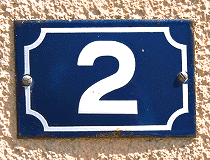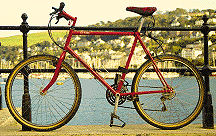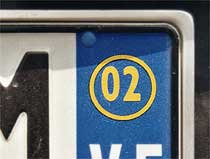# 2

2 is a prime number and is the only even prime number.

= 1 × 2
Factorial 2 or 2!

1, 1, 2, 3, 5, 8, 13...
A Fibonacci number.

A deuce, a couple, a brace, a duo or a pair...

There are two blades on a pair of scissors and two sides to a piece of paper. People have two hands and so do some clocks. There are two sexes and two sides to an argument. Two-dimensional means that something has just length and width, but no depth.

Two's company, three's a crowd all depends on who you happen to be with.

Bi- means two. For example, a bicycle has two wheels and a bigamist has two husbands or two wives.Two wheels on a bicycle.

In binary code numbers are written to the base two. It uses just two symbols, 0 and 1. The numbers 1, 2, 3, 4, 5, 6… become 1, 10, 11, 100, 101, 110…

The letters H, I and X all have two lines of symmetry.

Two has a very special property because
2 + 2 = 4
and also
2 × 2 = 4.

Try to find a number ? where
? + ? + ?
and
? × ? × ?
both give the same answer. A calculator could help you here.